# 44: Statistics and Patient Safety Flashcards Preview

## Absite > 44: Statistics and Patient Safety > Flashcards

Flashcards in 44: Statistics and Patient Safety Deck (32):
1

## Which statistical term is defined as the degree of closeness of measurements of a quantity to that quantity's true value?

### Accuracy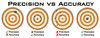2

## Which statistical tool estimates survival in small groups?

### Kaplan-Meier estimator[Wikipedia: The Kaplan–Meier estimator, also known as the product limit estimator, is a non-parametric statistic used to estimate the survival function from lifetime data. In medical research, it is often used to measure the fraction of patients living for a certain amount of time after treatment. In other fields, Kaplan–Meier estimators may be used to measure the length of time people remain unemployed after a job loss, the time-to-failure of machine parts, or how long fleshy fruits remain on plants before they are removed by frugivores. The estimator is named after Edward L. Kaplan and Paul Meier, who each submitted similar manuscripts to the Journal of the American Statistical Association. The journal editor, John Tukey, convinced them to combine their work into one paper, which has been cited about 34,000 times since its publication.]3

4

## Which statistical term is defined as the probability of making the correct conclusion (equals 1 - probability of a type II error)?

### Power[Likelihood that the conclusion of the test is true. Larger sample size increases power of a test.][Wikipedia: The power or sensitivity of a binary hypothesis test is the probability that the test correctly rejects the null hypothesis (H0) when the alternative hypothesis (H1) is true. It can be equivalently thought of as the probability of accepting the alternative hypothesis (H1) when it is true—that is, the ability of a test to detect an effect, if the effect actually exists. That is,The power of a test sometimes, less formally, refers to the probability of rejecting the null when it is not correct, though this is not the formal definition stated above. The power is in general a function of the possible distributions, often determined by a parameter, under the alternative hypothesis. As the power increases, there are decreasing chances of a Type II error (false negative), which are also referred to as the false negative rate (β) since the power is equal to 1−β, again, under the alternative hypothesis. A similar concept is Type I error, also referred to as the “false positive rate” or the level of a test under the null hypothesis.Power analysis can be used to calculate the minimum sample size required so that one can be reasonably likely to detect an effect of a given size. For example: “how many times do I need to toss a coin to conclude it is rigged?” Power analysis can also be used to calculate the minimum effect size that is likely to be detected in a study using a given sample size. In addition, the concept of power is used to make comparisons between different statistical testing procedures: for example, between a parametric and a nonparametric test of the same hypothesis.]5

## What does p < 0.05 mean?

### There is > 95% chance that the difference between the populations is true and that there is < 5% likelihood that the difference is not true and occured by chance alone6

## Which type of study/trial compares a population with a disease with a similar population without the disease and looks for the frequency of a risk factor between the groups?

### Case-control study[Must be retrospective.]7

## Which type of error is defined as the incorrect rejection of the null hypothesis (falsely assumed there was a difference when no difference exists)?

### Type I error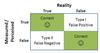8

9

## Which type of study/trial combines data from different studies?

### Meta-analysis10

## Which statistical tool compares the means between 2 independent groups?

### Student's t test[Variable is quantitative.][Wikipedia: A t-test is any statistical hypothesis test in which the test statistic follows a Student's t-distribution under the null hypothesis. It can be used to determine if two sets of data are significantly different from each other.A two-sample location test of the null hypothesis such that the means of two populations are equal. All such tests are usually called Student's t-tests, though strictly speaking that name should only be used if the variances of the two populations are also assumed to be equal.The independent samples t-test is used when two separate sets of independent and identically distributed samples are obtained, one from each of the two populations being compared. For example, suppose we are evaluating the effect of a medical treatment, and we enroll 100 subjects into our study, then randomly assign 50 subjects to the treatment group and 50 subjects to the control group. In this case, we have two independent samples and would use the unpaired form of the t-test. The randomization is not essential here – if we contacted 100 people by phone and obtained each person's age and gender, and then used a two-sample t-test to see whether the mean ages differ by gender, this would also be an independent samples t-test, even though the data is observational.]11

## What is the JCAHO definition of a sentinel event?

### Unexpected occurrence involving death or serious injury, or the risk thereof[Hospital undergoes root cause analysis to prevent and minimize future occurrences (eg wrong site surgery).]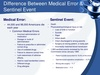12

## Which statistical term is defined as the number of people with disease in a population (eg the number of patients in United States with colon cancer)?

### Prevalence[Longstanding disease increases prevalence.]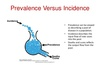13

## Which statistical term is defined as the degree to which repeated measurements under unchanged conditions show the same results?

### Precision14

## What is variance?

### Spread of data around a mean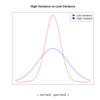15

16

## Which kind of variables do nonparametric statistics compare?

### Categorical (qualitative) variables[I.E. race, sex, medical problems, diseases, medications.]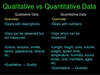17

## Which statistical term is defined as the likelihood that with a positive result, the patient actually has the disease [true positives/(true positives + false positives)]?

### Positive predictive value (PPV)[Wikipedia: Note that the PPV is not intrinsic to the test—it depends also on the prevalence. Due to the large effect of prevalence upon predictive values, a standardized approach has been proposed, where the PPV is normalized to a prevalence of 50%. PPV is directly proportional to the prevalence of the disease or condition. In the above example, if the group of people tested had included a higher proportion of people with bowel cancer, then the PPV would probably come out higher and the NPV lower. If everybody in the group had bowel cancer, the PPV would be 100% and the NPV 0%.To overcome this problem, NPV and PPV should only be used if the ratio of the number of patients in the disease group and the number of patients in the healthy control group used to establish the NPV and PPV is equivalent to the prevalence of the diseases in the studied population, or, in case two disease groups are compared, if the ratio of the number of patients in disease group 1 and the number of patients in disease group 2 is equivalent to the ratio of the prevalences of the two diseases studied. Otherwise, positive and negative likelihood ratios are more accurate than NPV and PPV, because likelihood ratios do not depend on prevalence.When an individual being tested has a different pre-test probability of having a condition than the control groups used to establish the PPV and NPV, the PPV and NPV are generally distinguished from the positive and negative post-test probabilities, with the PPV and NPV referring to the ones established by the control groups, and the post-test probabilities referring to the ones for the tested individual (as estimated, for example, by likelihood ratios). Preferably, in such cases, a large group of equivalent individuals should be studied, in order to establish separate positive and negative predictive values for use of the test in such individuals.]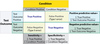18

## Which statistical term is defined as the ability to state no disease is present [true negatives/(true negatives + false positives)]?

### Specificity[Indicates the number of people who do not have the disease who test negative. With high specificity, a positive test result means a patient is very likely to have disease. Sensitivity and specificity are independent of disease prevalence.]19

## Which statistical term is defined as the number of new cases diagnosed over a certain time frame in a population (eg the number of patients in the US with newly diagnosed colon cancer in 2003)?

### Incidence[Wikipedia: Incidence in epidemiology is a measure of the probability of occurrence of a given medical condition in a population within a specified period of time. Although sometimes loosely expressed simply as the number of new cases during some time period, it is better expressed as a proportion or a rate with a denominator.Incidence proportion (also known as cumulative incidence) is the number of new cases within a specified time period divided by the size of the population initially at risk. For example, if a population initially contains 1,000 non-diseased persons and 28 develop a condition over two years of observation, the incidence proportion is 28 cases per 1,000 persons per two years, i.e. 2.8%.]20

## Which type of study/trial compares disease rates between exposed and unexposed groups (non-random assignment)?

### Cohort study[Typically a prospective study but can be retrospective.]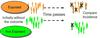21
22

## Which statistical tool is used to compare two population means where you have two samples in which observations in one sample can be grouped with observations in the other sample?

### paired t-test[Variable is quantitative. Before and after studies (eg weight before and after, drug vs placebo).][Wikipedia: A test of the null hypothesis that the difference between two responses measured on the same statistical unit has a mean value of zero. For example, suppose we measure the size of a cancer patient's tumor before and after a treatment. If the treatment is effective, we expect the tumor size for many of the patients to be smaller following the treatment. This is often referred to as the "paired" or "repeated measures" t-test.A typical example of the repeated measures t-test would be where subjects are tested prior to a treatment, say for high blood pressure, and the same subjects are tested again after treatment with a blood-pressure lowering medication. By comparing the same patient's numbers before and after treatment, we are effectively using each patient as their own control. That way the correct rejection of the null hypothesis (here: of no difference made by the treatment) can become much more likely, with statistical power increasing simply because the random between-patient variation has now been eliminated. Note however that an increase of statistical power comes at a price: more tests are required, each subject having to be tested twice. Because half of the sample now depends on the other half, the paired version of Student's t-test has only "n/2–1" degrees of freedom (with n being the total number of observations)[citation needed]. Pairs become individual test units, and the sample has to be doubled to achieve the same number of degrees of freedom.A paired samples t-test based on a "matched-pairs sample" results from an unpaired sample that is subsequently used to form a paired sample, by using additional variables that were measured along with the variable of interest. The matching is carried out by identifying pairs of values consisting of one observation from each of the two samples, where the pair is similar in terms of other measured variables. This approach is sometimes used in observational studies to reduce or eliminate the effects of confounding factors.Paired samples t-tests are often referred to as "dependent samples t-tests".]23

24

## Which type of error is defined as the incorrect acceptance of the null hypothesis (falsely assumes there is no difference when a difference exists)

### Type II error[Can occur due to a small sample size.]25

## Which statistical term is defined as the ability to detect disease [true positives/(true positives + false negatives)]?

### Sensitivity[Indicates the number of people who have the disease who test positive. With high sensitivity, a negative test result means a patient is very unlikely to have disease. Sensitivity and specificity are independent of disease prevalence.]26

## Which statistical term is defined as the likelihood that with a negative result, the patient does not have the disease [true negatives/(true negatives + false negatives)]?

### Negative predictive value (NPV)[Wikipedia: Note that the PPV is not intrinsic to the test—it depends also on the prevalence. Due to the large effect of prevalence upon predictive values, a standardized approach has been proposed, where the PPV is normalized to a prevalence of 50%. PPV is directly proportional to the prevalence of the disease or condition. In the above example, if the group of people tested had included a higher proportion of people with bowel cancer, then the PPV would probably come out higher and the NPV lower. If everybody in the group had bowel cancer, the PPV would be 100% and the NPV 0%.To overcome this problem, NPV and PPV should only be used if the ratio of the number of patients in the disease group and the number of patients in the healthy control group used to establish the NPV and PPV is equivalent to the prevalence of the diseases in the studied population, or, in case two disease groups are compared, if the ratio of the number of patients in disease group 1 and the number of patients in disease group 2 is equivalent to the ratio of the prevalences of the two diseases studied. Otherwise, positive and negative likelihood ratios are more accurate than NPV and PPV, because likelihood ratios do not depend on prevalence.When an individual being tested has a different pre-test probability of having a condition than the control groups used to establish the PPV and NPV, the PPV and NPV are generally distinguished from the positive and negative post-test probabilities, with the PPV and NPV referring to the ones established by the control groups, and the post-test probabilities referring to the ones for the tested individual (as estimated, for example, by likelihood ratios). Preferably, in such cases, a large group of equivalent individuals should be studied, in order to establish separate positive and negative predictive values for use of the test in such individuals.]27

28

## Which statistical term is defined as the incidence in exposed/incidence in unexposed?

### Relative risk[Wikipedia: In statistics and epidemiology, relative risk or risk ratio (RR) is the ratio of the probability of an event occurring (for example, developing a disease, being injured) in an exposed group to the probability of the event occurring in a comparison, non-exposed group. Relative risk includes two important features: (i) a comparison of risk between two "exposures" puts risks in context, and (ii) "exposure" is ensured by having proper denominators for each group representing the exposure.]29

## Which statistical tool compares 2 groups with categorical (qualitative) variables?

### Chi-squared test[I.E. # of obese patients with and without diabetes vs number of nonobese patients with and without diabetes.][Wikipedia: A chi-squared test, also written as χ2 test, is any statistical hypothesis test wherein the sampling distribution of the test statistic is a chi-squared distribution when the null hypothesis is true. Without other qualification, 'chi-squared test' often is used as short for Pearson's chi-squared test.Chi-squared tests are often constructed from a sum of squared errors, or through the sample variance. Test statistics that follow a chi-squared distribution arise from an assumption of independent normally distributed data, which is valid in many cases due to the central limit theorem. A chi-squared test can be used to attempt rejection of the null hypothesis that the data are independent.Also considered a chi-squared test is a test in which this is asymptotically true, meaning that the sampling distribution (if the null hypothesis is true) can be made to approximate a chi-squared distribution as closely as desired by making the sample size large enough. The chi-squared test is used to determine whether there is a significant difference between the expected frequencies and the observed frequencies in one or more categories.]30

## What is mode, mean, and median?

### Mode: Most frequently occurring value Mean: Average of all values Median: Middle value of a set of data31

32